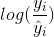# How does log of y and y_pred make a RMSPE to RMSE?

This is from the notes on lesson 6, but basically it says

Can someone show and explain to me the math that does this? This image is from https://github.com/hiromis/notes/blob/master/Lesson6.md

4 Likes

The way Jeremy said it in the lecture seems to be wrong. One can see this with a simple example, by choosing to have only one pair of (y,y^) to compare (so n=1 in the equation above):

Maybe @jeremy can clarify this?

EDIT: Wait, mistake in the formula
EDIT^2: Fixed

1 Like

I have the same question. The summands in RMSE_LOG can merely be rewritten aswhich is still not the same as the RMSPE mathematically. The fact that log() turns quotients into differences and vice-versa does not seem to be enough in this case.

Yes same question here.

I have the same question as well.

Same question as well.

We need to find a function mapping for y and y_pred that will create new Y and Y_PRED s.t that the RMSPE (y,y_pred) = RMSE(Y,Y_PRED). This function comes out to be a log function using Taylor’s expansion. Please follow the link below for more: